# Physics Topic-wise Test - 1

## 45 Questions MCQ Test NEET Mock Test Series & Past Year Papers | Physics Topic-wise Test - 1

Description
This mock test of Physics Topic-wise Test - 1 for NEET helps you for every NEET entrance exam. This contains 45 Multiple Choice Questions for NEET Physics Topic-wise Test - 1 (mcq) to study with solutions a complete question bank. The solved questions answers in this Physics Topic-wise Test - 1 quiz give you a good mix of easy questions and tough questions. NEET students definitely take this Physics Topic-wise Test - 1 exercise for a better result in the exam. You can find other Physics Topic-wise Test - 1 extra questions, long questions & short questions for NEET on EduRev as well by searching above.
QUESTION: 1

### The Dimension of σb4  (σ = Stefan's constant and b = Wein's Constant) are :

Solution:

Surface tension = Force/Length = newton/metre.

QUESTION: 2

### An athlete completes one round of a circular track of radius R in 40 seconds. The displacement at the end of 2 minutes 20 seconds is

Solution:

2 min 20 second = 140 second.
he completed one round in 40 second.
in 140 second, he will cover= 140/40=3.5 round.
after 3.5 round, he would be on the opposite side of the starting point.
So the displacement will be the diameter or 2R.

QUESTION: 3

### A body slides down a smooth curved track which is one quadrant of a circle having radius 10 m. The speed of the body at the bottom of the track is: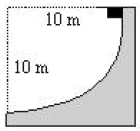Solution: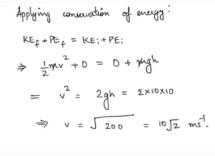QUESTION: 4

A particle of mass m is driven by a machine that delivers a constant power k watts. If the particle starts from rest the force on the particle at time t is

Solution:

Constant power acting on the particle of mass m is k watt.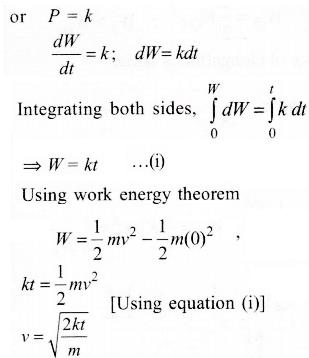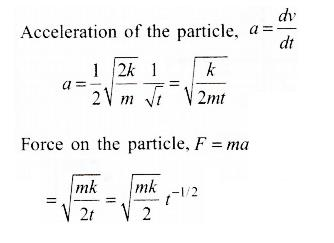QUESTION: 5

An engine pumps up 100 kg of water through a height of 10m in 5 sec then if the efficiency of the engine os 60% then calculate the power of the engine where g = 10m/s

Solution:

Output power = mgh/t
= 100 kg × 10 m/s² × 10 m / 5 s
= 2 × 10³ Watt
= 2 kW

Effeciency = [(Output power) / (Input power)] × 100
60 = [2 kW / (Input power)] × 100
Input power = 3.33 kW

Power of engine is 3.33 kW

QUESTION: 6

A block of mass 4 kg is placed on a rough horizontal plane. A time dependent force F = k t2 acts on the block, where k = 2 N/s2 Force of friction between block and the plane at t = 2 sec (given coefficient of friction is 0.3)

Solution:

F = kt2

F = 2t2 --- k = 2

Fmax = μmg

= (0.8) (4) (10)

= 32 N

applied force = F = 2t2

at t = 2s,  F = 2 (2)2 = 8N

Hence body cannot move.

And force of friction between block and plane = applied farce = 8 N

QUESTION: 7

A particle collides with another particle such that the second particle starts moving and the first particle stops just after the collision. Then which of the following conditions must always be valid? (m/M = ratio of masses)

Solution:

It m = m and mu, + mu2 + o = mv
mu2 = m (v - u1)
m/m = v - u1/u2 ≤ 1

QUESTION: 8

A sphere has a perfectly elastic oblique collision with another identical sphere, which is initially at rest. The angle between their direction after the collision is

Solution:
QUESTION: 9

Two bodies having masses in the ratio of 3 : 1 possess the same kinetic energy. The ratio of their linear momentum is

Solution:

P = √2 mk
P1/p2 = √3/1

QUESTION: 10

The equation of a wave is given by y = Asin ω(x/v-K) Where Asin ω is angular velocity and v is linear velocity. The dimension of K is

Solution:

Check the dimensional correctness -

L.H.S.=R.H.S.

- wherein

It is based on the principle of homogeneity. According to this principle both sides of an equation must be the same.
From principle of homogeneity (x/v) has dimension of T.

QUESTION: 11

If two numerically equal forces P & P acting at a point produce a resultant force of magnitude P itself, then the angle between the two original forces is

Solution:

Since magnitude of all vectors is same let it be R then : R2=R2+ R2+2R×Rcosθ ; R2=2R2+2R2cosθ
Solving equation we get cosθ=(-1/2) ; θ=120 degrees

QUESTION: 12

A physical quantity X is related to four observables a,b,c & d as follows X = a3b2/d√c. The percentage error of measurement in a,b,c and d are 1%,3%,4% & 2% respectively, the percentage error in the quantity X is

Solution:

Relative error in P is given by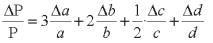So, percentage error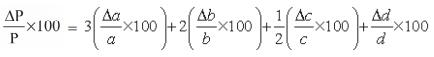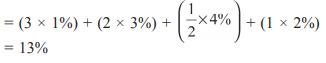Rounded off value of P = 3.8.

QUESTION: 13

A particle moving in a straight line covers half the distance with speed of 3m/s. The other half of the distance is covered in two equal time intervals with speed of 4.5 m/s 7.5m/s respectively. The average speed of the particle during this motion is

Solution: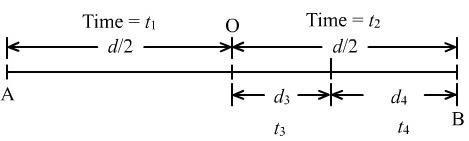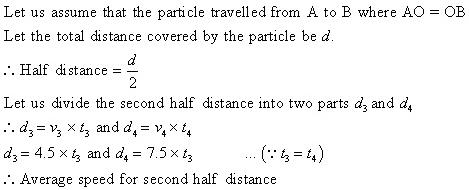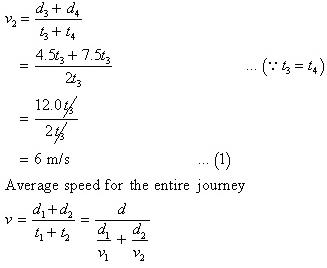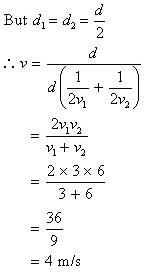QUESTION: 14

A particle moves along a straight line such that its displacement at any time is given by s = t3 - 6t2 + 3t + 4 meters. The velocity when the acceleration is zero is

Solution:

v = 3t2 - 12 - 24 + 3
a = 6t - 12
t = 2
v = 12 - 24  + 3
= -9

QUESTION: 15

Three blocks of masses M1,M& M3 are connected by mass-less strings as shown on a friction-less table. They are pulled with a force T3 = 40N. If M1 = 10Kg, M2 = 6Kg, M3 = 4KKg   then Tension T2 will be: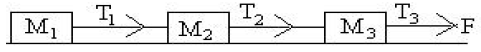Solution:

For equilibrium of all 3 masses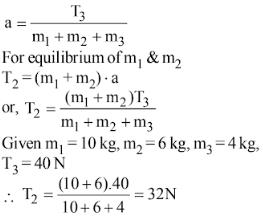QUESTION: 16

The blocks of mass M1 and M2 are connected to each other through a light spring as shown in figure. If we push mass M1 with a force of F and cause acceleration a in mass M1, what will be the acceleration in M2: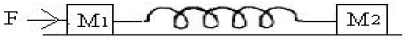Solution:QUESTION: 17

A balloon has 5gm of air. A small hole is pierced into it, the air escapes at a uniform rate with a velocity of 4cm/sec. If the balloon shrinks completely in 2.5 second, the mean force acting on the balloon is

Solution: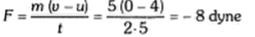QUESTION: 18

Two skaters of masses 40 kg and 60 kg respectively stand facing each other at S1 and S2 where S1S2 is 5m. They pull on a massless rope stretched between them, then they meet at

Solution:

d = m2d/m1 + m2
d = 3 meters.

QUESTION: 19

A man sits on a chair supported by a rope passing over a friction less fixed pulley. The man who weights 1000N exerts a force of 450 N on the chair downwards while pulling on the rope. If the chair weight 250 N and g is 10 ms-2, then the acceleration of the chair is

Solution:

Mass of man = 100 kg,
Mass of chair = 25 kg.
a = ?
For chair:
T - 450 - 250 = 25 a.
For man:
T + 450 - 1000 = 100a.
a = 2m/s2, T = 750 N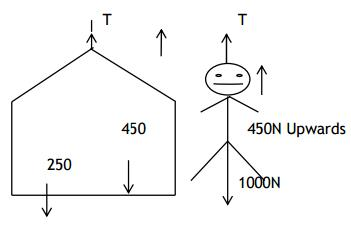QUESTION: 20

A block of mass 4 kg is suspended through two light spring balances A and B. Then A and B will read __respectively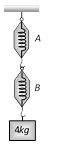Solution:

As the spring balances are massless therefore the reading of both balance should be equal.

QUESTION: 21

In a rocket of mass 1000 kg fuel is consumed at a rate of 40 kg/s.The velocity of the gases ejected from the rocket is 5 x 104 m/s.The thrust on the rocket is

Solution:

Thurst = 5 x 104 x 40

= 2 x 106 N.

QUESTION: 22

Which of the following statements is false ?

Solution:

F = uN
which depends on roughness.

QUESTION: 23

A man walks over a rough surface; the angle between force of friction and the instantaneous velocity of the person is

Solution:

Force is directed along the direction of motion.

QUESTION: 24

A heavy uniform chain lies on a horizontal table top. If the coefficient of friction between the chain and the table surface is 0.25, then the maximum fraction of the length of the chain, that can hang over one edge of the table is

Solution:

nL×100/(1+n)L =25/1.25 =20%

QUESTION: 25

A particle is projected along the line of greatest slope up a rough plane inclined at an angle of 45°  with the horizontal. If the coefficient of friction is 1/2, their retardation is

Solution: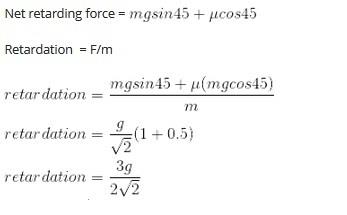QUESTION: 26

A ball thrown vertically upward returns to its starting point in 4s. Its initial speed is

Solution:

V = u + at
o = u - 9.8x2
u = 19.6

QUESTION: 27

A stone is dropped from a certain height which can reach the ground in 5 second. If the stone is stopped after 3 second of its fall and then allowed to fall again, then the time taken by the stone to reach the ground for the remaining distance is

Solution: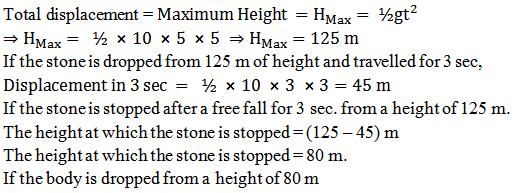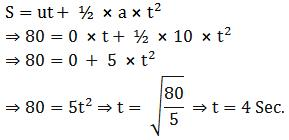QUESTION: 28

A stone is projected upwards from the top of a building with some initial speed and reaches the ground in 5sec. Now it is allowed to fall with the same initial speed downwards and reaches the ground in 3 sec. If the stone is allowed to fall freely under gravity from the same point, it will reach the ground in

Solution:
QUESTION: 29

The position of a projectile is given by x = v0t - Kt2 where v0 equals 250 m/s and t is measured in seconds. Given that the velocity of the projectile falls to a value of 150 m/s at t = 5s. The value of the K is

Solution:

x = Vot - Kt2
v = V0 - 2kt
150 = 250 - 2k x 5
= k = 10.

QUESTION: 30

The two ends of a train moving with a constant acceleration pass a certain point with velocities u and v. The velocity with which the middle point of the train passes the same point is

Solution:

Let the length of the train be ‘L’.

As it crosses the point, the initial velocity can be taken as ‘u’ and final velocity as ‘v’.

So, v2 = u2 + 2aL

=> v2 – u2 = 2aL ……………(1)

Suppose the velocity with which the midpoint crosses that certain point be v/. In this case the distance traveled is L/2.

So,

(v/)2 = u2 + 2a(L/2)

=> (v/)2 = u2 + ½ × (v2 – u2) [using (1)]

=> (v/)2 = (v2 + u2)/2

=> v/ = {(v2 + u2)/2}1/2

This is the velocity of the midpoint of the train with which it crosses that point.

QUESTION: 31

The sum & differences of two perpendicular vectors of equal magnitudes are

Solution: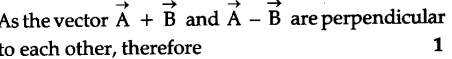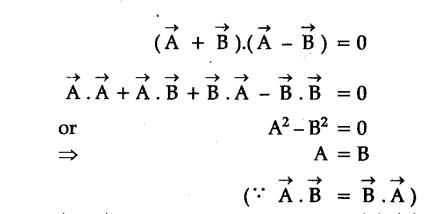QUESTION: 32

An aeroplane is moving on a circular path with a speed of 250 kmph. The change in velocity (in kmph) in half revolution is

Solution: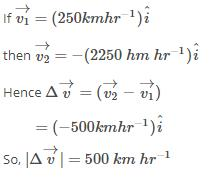QUESTION: 33

If the sum of two unit vectors is also a unit vector, then the magnitude of their difference is

Solution: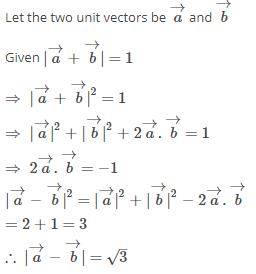QUESTION: 34

A person aims a gun at a bird from a point, at a horizontal distance of 100 m.If gun can impart a speed of 500m/s to the bullet,at what height above the bird must he aim his gun in order to hit it? (g = 10 ms-2)

Solution:

From given the data
Range R = 2 ⨯100 m = 200 m
Initial velocity u = 500 m/s
We know that Range R =

Horizontal distance x = 100 m
Velocity v = 500 m.s-1
Time taken to travel this distance t = x/v = 100/500 = 1/5s
Vertical distance travelled by the bullet in time 1/5s is given by,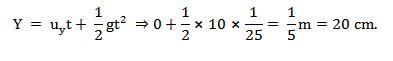If the person directly aims at the bird, the bullet will hit 20 cm below
the bird. Thus the gun must be aimed at 20 cm above the position of the bird.

QUESTION: 35

The hight y and distance x along the horizontal plane of a projectile on a certain planet (With no surrounding atmosphere) are given as y=y=8t-5t2 mtr. and x = 6t metres, where t is in seconds. The velocity with which the projectile is projected is

Solution:

Y = 8t - 5t²
differentiate wrt time
dy/dt = 8 -10t

we know, change in position per unit time is called , velcity .
e.g dy/dt = Vy

Vy = 8 -10t
now at t = 0 ( initially )
Vy =8 -10× 0 = 8

x = 6t
differentiate wrt time
dx/dt = 6
similarly , dx/dt = Vx =6

now,
V = Vx i + Vy j
= 8 i + 6 j

magnitude of velocity =| V | =√(8² + 6²) =10 m/s

QUESTION: 36

A helicopter flying at 20 m/s horizontally drops a rescue bag. Ignoring air resistance & taking g = 10ms-2, the displacement of the bag 5 seconds after the drop began is nearly

Solution:

Sx = 20 x 5
Sy = 1/2 x w x 25
S = √sx2 + sy2
= 160.

QUESTION: 37

When the angular velocity of a uniformly rotating body has increased thrice, the resultant of forces applied to it increase by 60 N. Find the acceleration of the body in the two cases. The mass of the body, m = 3kg

Solution: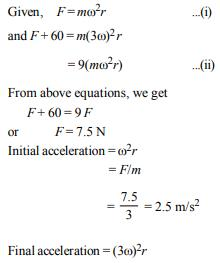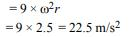QUESTION: 38

In case of uniform circular motion which of the following physical quantities do not remain constant?

Solution:

Though speed is constant but direction 0f motion and hence the velocity is variable. Therefore,the momentum is not constant

QUESTION: 39

A parachutist of weight W strikes the ground with his legs fixed and comes to rest with an upward acceleration of magnitude 3g . force exerted on him by ground during landing is

Solution:

m = w/g

3mg = n - w

N = 4mg

= 4w

QUESTION: 40

An unloaded car moving with velocity u on a road can be stopped in a distance s. If passengers add 40% to its weight and braking force remains the same, the stopping distance at velocity u is now

Solution: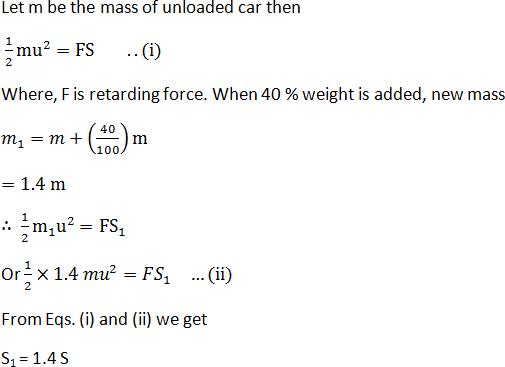QUESTION: 41

A 15 kg mass is accelerated from rest with a force of 100 N. As it moves faster, friction & air resistance create an oppositely directed retarding force given by FR = A+BV. where A=25 N and B= 0.5 Ns/milter. At what velocity does the acceleration equal to one half of the initial acceleration ?

Solution:
QUESTION: 42

The minimum acceleration that must be imparted to the cart in figure given so that the block A will bot fall, given µ is the coefficient of friction between the surface of block and cart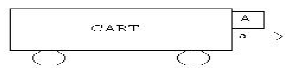Solution:

uN = mg
N = ma
u ma = mg

a = g/u

QUESTION: 43

A satellite in force-free space sweeps stationary interplanetary dust at a rate (dM/dt) = αv. The acceleration of satellite is

Solution:

F = αv2
a = -αv2/M

QUESTION: 44

In the expression for Boyle’s law, the product ‘PV’ has dimensions of

Solution: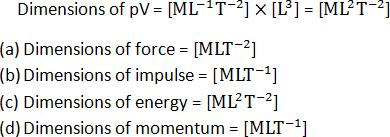QUESTION: 45

The rear side of a truck is open and a box of 40 kg mass is placed 5m away from the open end as shown in fig. The coefficient of friction between the box and the surface below it is 0.15. On a straight road, the truck starts from rest and accelerates with 2ms-2 . At what distance from the starting point does the box fall off the truck.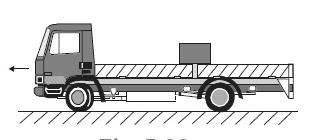Solution:

Mass of the box, m = 40kg

Coefficient of friction, µ = 0.15

Initial velocity, u = 0

Acceleration, a = 2 m/s2

Distance of the box from the end of the truck, s' = 5m

As per Newton's second law of motion, the force on the box caused by the acceleration motion of the truck is given by:

F = ma

= 40 x 2 = 80N

As per Newton's third law of motion, a reaction force of 80 N is acting on the boxin the backward direction. The backward motion of the box is opposed by the force of friction f, acting between the box and the floor of the turck. This force is given by:

f = μmg

= 0.15 x 40 x 10 = 60N

∴Net force acting on the block:

Fnet = 80 - 60 = 20N backward

The backward acceleration produced in the box is given by aback = Fnet/m = 20/40 = 0.5 ms-2

Using the second equation of motion, time t can be calculated as :

s' = ut + (1/2)abackt2

5 = 0 + (1/2) x 0.5 x t2

∴ t = √20s

Here the box fall from the truck after √20s from start.

The distance s, travelled by the truck in √20s is given by the relation:

s = ut + (1/2)at2

= 0 + (1/2) x 2 x (√20)2

= 20 m